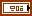Plane Property

FlexRIO Help

Edition Date: November 2015

Part Number: 372614J-01

»View Product Info

Short Name: Plane

Requires: Full Development System

To Use: Create a property.

Reads or writes the four coefficients of the plane equation.

Ax+By+Cz+D=0

Elements

Name Description
A The value of the A coefficient of the plane equation.
B The value of the B coefficient of the plane equation.
C The value of the C coefficient of the plane equation.
D The value of the D coefficient of the plane equation.

Remarks

The following table lists the characteristics of this property.

 Data typePermissions Read/Write Available in Run-Time Engine Yes Available in Real-Time Operating System No Settable when the VI is running Yes Loads the front panel into memory No Need to authenticate before use No Loads the block diagram into memory No Remote access allowed Yes